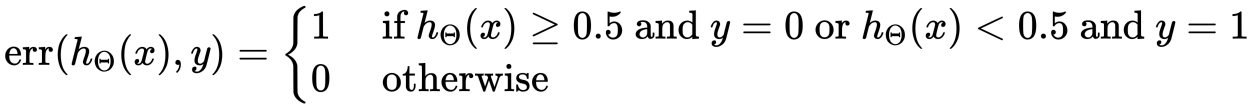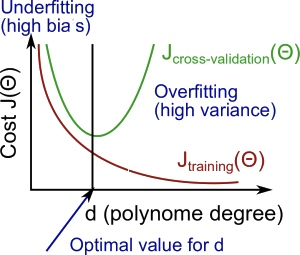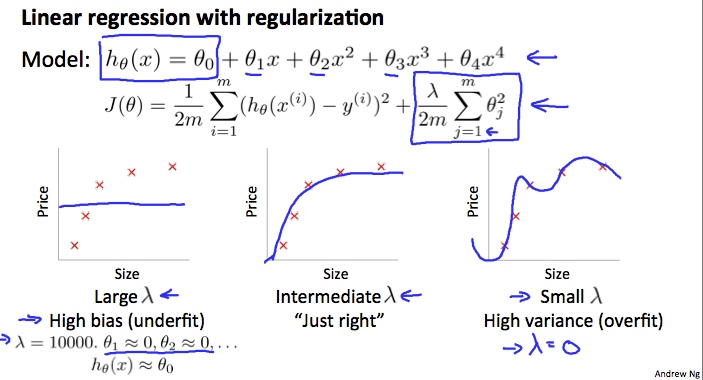## 1. 评估一个学习算法

### 1.1 决定下一步应该做什么

• 获得更多的训练样本
• 尝试缩小特征矩阵
• 尝试获得额外特征
• 尝试增加多项式特征
• 尝试减小$\lambda$
• 尝试增大$\lambda$

### 1.2 评估一个假设

• 利用训练集学习参数$\theta$并最小化训练集误差$J_{train}(\theta)$
• 计算测试集误差$J_{test}(\theta)$

（1）对于线性回归：

$J_{\text {test}}(\Theta)=\frac{1}{2 m_{t e s t}} \sum_{i=1}^{m_{t e s t}}\left(h_{\Theta}\left(x_{\text {test}}^{(i)}\right)-y_{t e s t}^{(i)}\right)^{2}$

（2）对于分类问题，首先定义分类误差函数：$\text { Test Error }=\frac{1}{m_{\text {test}}} \sum_{i=1}^{m_{\text { test }}} \operatorname{err}\left(h_{\Theta}\left(x_{\text {test}}^{(i)}\right), y_{\text {test}}^{(i)}\right)$

### 1.3 模型选择和训练/验证/测试数据集

• 训练集：60%
• 交叉验证集：20%
• 测试集：20%

（1）对于每一个多项式（假设函数）利用训练集优化参数$\Theta$
（2）利用交叉验证集找到具有最小损失的多项式程度d（假设函数）；
（3）利用测试集衡量泛化误差。

## 2. 偏差与方差

### 2.1 诊断偏差与方差### 2.2 正则化与偏差/方差（1）建立一个$\lambda$的列表($ie.\lambda \in {0,0.01,0.02,0.04,0.08,0.16,0.32,0.64,1.28,2.56,5.12,10.24}$)；
（2）建立一个包含不同多项式级别和其他变量的模型集合；
（3）对不同的$\lambda$进行迭代，对于每个$\lambda$训练所有模型的$\Theta$
（4）利用学习到的参数$\Theta$计算不包含正则化项（$\lambda=0$）的交叉验证误差$J_{CV}(\Theta)$
（5）使用最好的

### 2.3 学习曲线

• 随着训练集的增大，二次方函数的误差也会增大；
• 当训练集大小超过特定的值m的时候，误差会趋向于稳定。

（1）当偏差较高的时候：

• 训练数据集较小：导致训练误差$J_{train}(\Theta)$很小，$J_{CV}(\Theta)$很高。
• 训练数据集较大：$J_{train}(\Theta)$$J_{CV}(\Theta)$都很高，并且$J_{C V}(\Theta) \approx J_{t r a i n}(\Theta)$
当一个学习算法偏差较高的时候，增加训练集大小不会有太大的帮助。

（2）当方差较高的时候：

• 训练数据集较小：导致训练误差$J_{train}(\Theta)$很小，$J_{CV}(\Theta)$很高。
• 训练数据集较大：$J_{train}(\Theta)$随着训练数据集大小的提升而增大，$J_{CV}(\Theta)$逐渐减小，并且$J_{\text {train}}(\Theta)
如果一个学习算法的方差较高，增加训练集大小会有一定的帮助。

### 2.4 再次决定下一步要做什么

（1）前面提到的缩小误差的方法适用的场景如下：

• 获得更多的训练样本：适合高方差场景（过拟合）
• 尝试缩小特征矩阵：适合高方差场景（过拟合）
• 尝试获得额外特征：适合高偏差场景（欠拟合）
• 尝试增加多项式特征：适合高偏差场景（欠拟合）
• 尝试减小$\lambda$：适合高偏差场景（欠拟合）
• 尝试增大$\lambda$：适合高方差场景（过拟合）

（2）诊断神经网络

• 有更少参数的神经网络容易导致欠拟合，但也计算简单；
• 有更多参数的神经网络容易导致过拟合，并且计算复杂。（可以通过增加正则化参数解决这一问题）

（3）其他更加复杂的影响

• 低阶多项式（低模型复杂度）有较高的偏差和较低的方差。这种情况下拟合效果不理想。
• 高阶多项式（高模型复杂度）对于训练数据拟合效果很好，但是测试数据效果很糟糕。他们在训练数据上有较低的偏差，但是有较高的方差。
• 通常实际中，我们倾向于选择两者之间的多项式，有较好的泛化能力并且拟合效果很好。